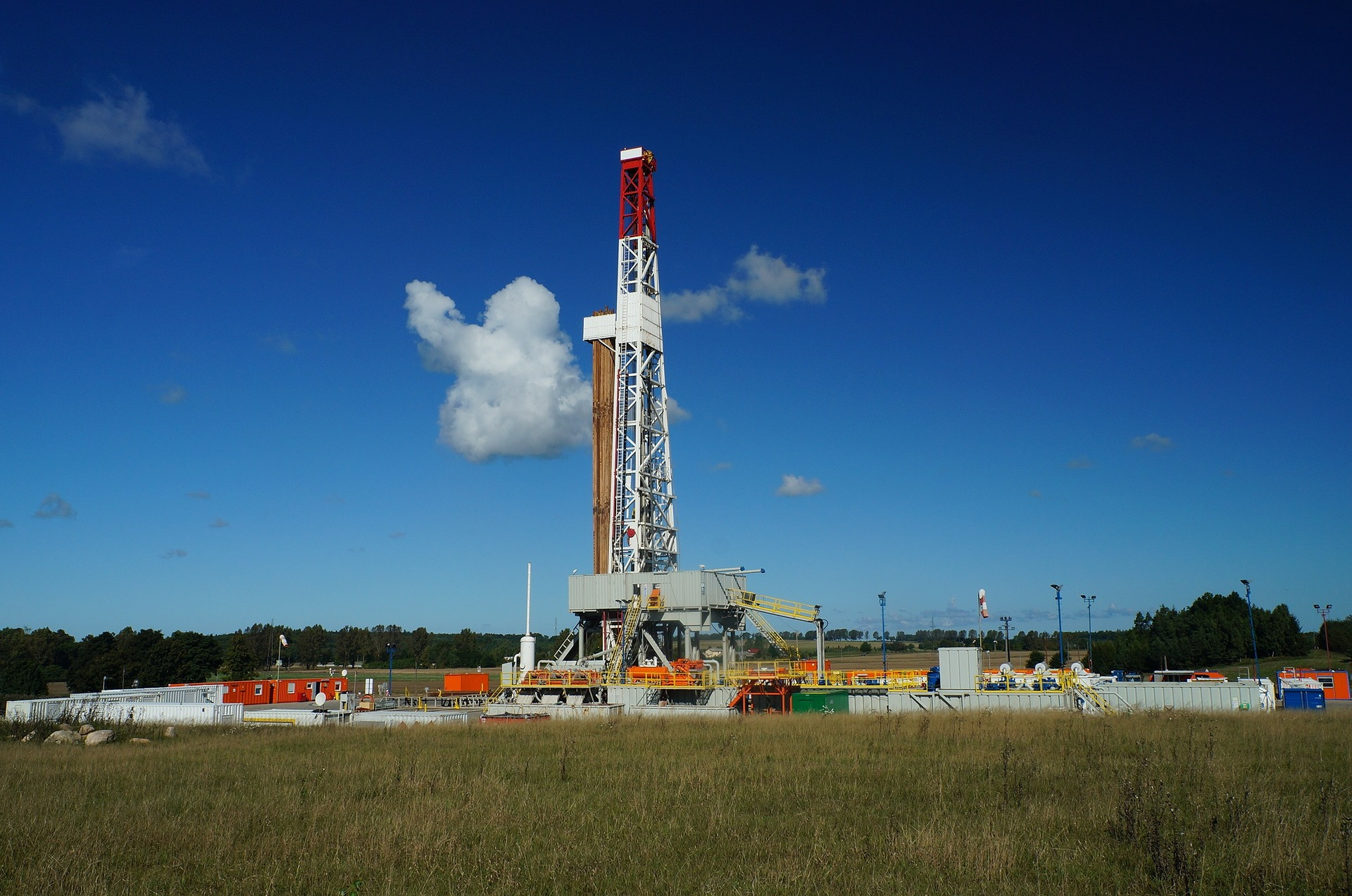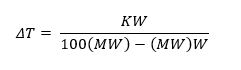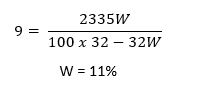# How to Estimate Hydrate Inhibitor to Depress Hydrate Formation

In previous post, I shared what is hydrate formation and primary conditions promoting hydrate formation. Quick review, hydrate in natural gas system is a physical combination of water and other small molecules to produce a solid which has an ice-like appearance. Hydrate should be control because it will cause several problems. The problems include restriction in flowlines, causing physical damage to chokes and instrumentation. One method to control hydrate formation is to inject hydrate inhibitor into production stream.

## How Hydrate Inhibitors Work

Hydrate inhibitor alters the chemical potential of the hydrate phase so that hydrate formation point is displaced to a lower temperature and/or a higher pressure.

## The Most Common Hydrate Inhibitors

The most common hydrate inhibitor used in field gas is methanol. It is relatively inexpensive. However, it will not be recovered and reused. Methanol is soluble in liquid hydrocarbons. If there is condensate in the stream, additional methanol is required because some of that methanol will dissolve in the condensate. Also, some of methanol vaporizes and goes into gas phase.

Ethylene glycol is the most common recoverable inhibitor. It is less soluble in hydrocarbon and has less vaporization loss than methanol.

## How to Calculate the Amount of Inhibitor Required

Suppose we have the following data:

• Shut-in bottom hole pressure     : 8000 psig
• Bottom hole temperature            : 224oF
• Initial flowing tubing pressure    : 4000 psig
• Flowing temperature                   : 65oF
• Condensate                                 : 60 bbl/MMscf

Composition and mole fraction of each component is as follow:

• Nitrogen                           0.0144
• Carbon dioxide                 0.0403
• Hydrogen sulfide              0.000019
• Methane                            0.8555
• Ethane                               0.0574
• Propane                             0.0179
• Isobutane                          0.0041
• n-Butane                           0.0041
• Pentane+                          0.0063

Assume that at the high flowing pressure there is no free water, but the gas is saturated.

## What is the required methanol to prevent hydrates from forming?

The step-by-step solution would be as follow.

 Determine water content and water condensed

The amount of water that will be condensed will be determined from figure below, assuming the gas is saturated at reservoir condition.

At 8000 psig and 224oF, water content is around 230 lb/MMscf.

At 4000 psig and 65oF, water content is around 10 lb/MMscf.

We can see that there is reduction of water content due to condensation of water.

The amount of water condensed is 230 – 10 = 220 lb/MMscf.

 Determine hydrate formation temperature

Because the composition is known, hydrate formation temperature will be predicted using vapor-solid equilibrium constant method.

Read more: Hydrate Prediction using Vapor-Solid Equilibrium Constant

By using vapor-solid equilibrium, we found that at 4000 psia and with composition as mentioned above, the hydrate formation temperature is approximately at 74oF.

 Calculate Required Dew Point Depression

Dew point depression is hydrate formation temperature minus operating temperature.

So, dew point depression is 74 – 65 = 9oF.

 Calculate Hydrate Inhibitor Required

The Hammerschmidt equation may be used to calculate the amount of inhibitor required in the water phase to lower the hydrate temperature. The equation is:Where:

ΔT           = depression of hydrate formation temperature, oF

MW       = molecular weight of inhibitor

K             = constant

W            = weight percent of inhibitor in final water phase

Molecular weight (MW) and constant (K) of several types of hydrate inhibitor is shown in table below.

 Inhibitor Molecular Weight (MW) Constant (K) Methanol 32.04 2335 Ethanol 46.07 2335 Isopropanol 60.10 2335 Ethylene glycol 62.07 2200 Propylene glycol 76.10 3590 Diethylene glycol 106.10 4370

Then, the concentration of methanol required in the liquid phase is:Calculate Desired Methanol in Liquid Phase

Desired methanol in liquid phase is concentration of methanol required in the liquid phase multiply by amount of water condensed (see Step 1).

So, 11% x 220 lb/MMscf = 24.2 lb/MMscf

 Estimate Amount of Methanol Flashed to The Vapor

We use graph below to estimate amount of methanol flashed to the vapor. It is a function of pressure and temperature.

At 4000 psia and 65oF:

So, methanol in vapor state = 1 x 11 = 11 lb/MMscf

 Calculate amount of methanol dissolved in Condensate

Solubility of methanol in condensate is about 0.5%. A barrel of hydrocarbon weighs about 300 lb. Therefore, the amount of methanol soluble in the hydrocarbon liquid phase will be:

0.5% x 300 lb/BBL x 60 BBL/MMscf = 90 lb/MMscf

 Calculate total amount of methanol

Total amount of methanol is summation of methanol in water liquid phase, vapor phase, and hydrocarbon liquid phase.

 Water liquid phase 24.2 lb/MMscf See Step 5 Vapor phase 11.0 lb/MMscf See Step 6 Hydrocarbon liquid phase 90.0 lb/MMscf See Step 7 Total 125.2 lb/MMscf

Density of methanol is approximately 6.6 lb/gal. So, 125.2 lb/MMscf is equivalent to 18.9 gal/MMscf.

Reference:

Arnold, Ken, and Maurice Stewart, “Surface Production Operations – Design of Oil Handling and Facilities” Vol 2 2nd Edition”, Elsevier, 1989.